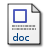# Proposed method for reconstructing velocity profiles using a multi-electrode electromagnetic flow meter

Kollar, László E., Lucas, Gary and Zhang, Zhichao (2014) Proposed method for reconstructing velocity profiles using a multi-electrode electromagnetic flow meter. Measurement Science and Technology, 25 (7). 075301. ISSN 0957-0233PDF - Accepted Version Download (7MB)Microsoft Word Restricted to Repository staff only Download (13MB)

## Abstract

An analytical method is developed for the reconstruction of velocity profiles using measured potential distributions obtained around the boundary of a multi-electrode electromagnetic flow meter (EMFM). The method is based on the discrete Fourier transform (DFT), and is implemented in Matlab. The method assumes the velocity profile in a section of a pipe as a superposition of polynomials up to sixth order. Each polynomial component is defined along a specific direction in the plane of the pipe section. For a potential distribution obtained in a uniform magnetic field, this direction is not unique for quadratic and higher-order components; thus, multiple possible solutions exist for the reconstructed velocity profile. A procedure for choosing the optimum velocity profile is proposed. It is applicable for single-phase or two-phase flows, and requires measurement of the potential distribution in a non-uniform magnetic field. The potential distribution in this non-uniform magnetic field is also calculated for the possible solutions using weight values. Then, the velocity profile with the calculated potential distribution which is closest to the measured one provides the optimum solution. The reliability of the method is first demonstrated by reconstructing an artificial velocity profile defined by polynomial functions. Next, velocity profiles in different two-phase flows, based on results from the literature, are used to define the input velocity fields. In all cases, COMSOL Multiphysics is used to model the physical specifications of the EMFM and to simulate the measurements; thus, COMSOL simulations produce the potential distributions on the internal circumference of the flow pipe. These potential distributions serve as inputs for the analytical method. The reconstructed velocity profiles show satisfactory agreement with the input velocity profiles. The method described in this paper is most suitable for stratified flows and is not applicable to axisymmetric flows in its present form. Its novelty is that it provides not only a mean flow velocity, but a velocity distribution in a circular pipe section as an analytical function of the spatial coordinates.

Item Type: Article T Technology > T Technology (General)T Technology > TA Engineering (General). Civil engineering (General) School of Computing and EngineeringSchool of Computing and Engineering > Systems Engineering Research Group Author Sara Taylor 29 May 2014 13:26 28 Aug 2021 19:10 https://eprints.hud.ac.uk/id/eprint/20419View Item